0

# 贝叶斯自举法Bayesian Bootstrap

“自举”（翻译自bootstrap）这个词汇在多个领域可能见到，它字面意思是提着靴子上的带子把自己提起来，这当然是不可能的，在机器学习领域可以理解为原样本自身的数据再抽样得出新的样本及统计量，也有被翻译为自助法的。Bayesian Bootstrap是一个强大的方法，它比其他的自举法更快，并且可以给出更紧密的置信区间，并避免许多极端情况。在本文中我们将详细地探讨这个简单但功能强大的过程。

## 自举

• 从样本{Xᵢ}ᵢⁿ中替换{X̃ᵢ}ᵢⁿ的n个观察样本。
• 计算估计量θ̂-bootstrap(X̃)。
• 大量重复步骤1和步骤2。

bootstrap仅仅是加权吗?

 from src.utils import *

N = 10_000
np.random.seed(1)
bootstrap_weights = np.random.multinomial(N, np.ones(N)/N)
np.sum(bootstrap_weights)

#结果：10000


 sns.countplot(bootstrap_weights, color='C0').set(title='Bootstrap Weights');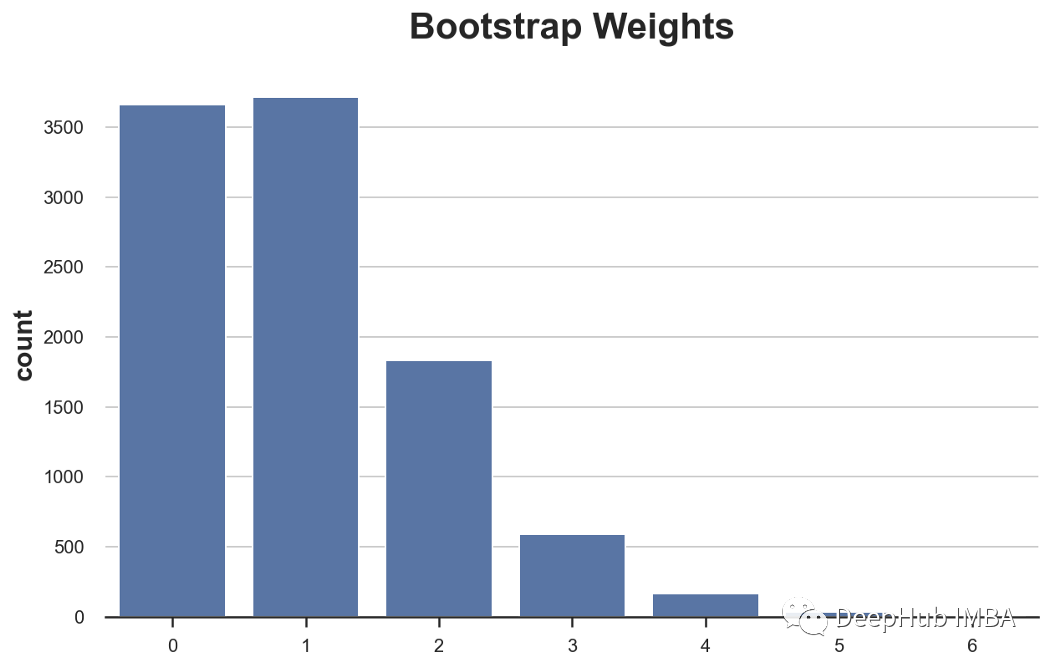## 贝叶斯自举

Bayesian bootstrap是由Rubin(1981)提出的，它基于一个非常简单的想法:为什么不画一个更平滑的权重分布?多项式分布的连续等价是狄利克雷分布。下面的图绘制了一次观测的多项和狄利克雷权重的概率分布(它们分别是泊松分布和伽马分布)。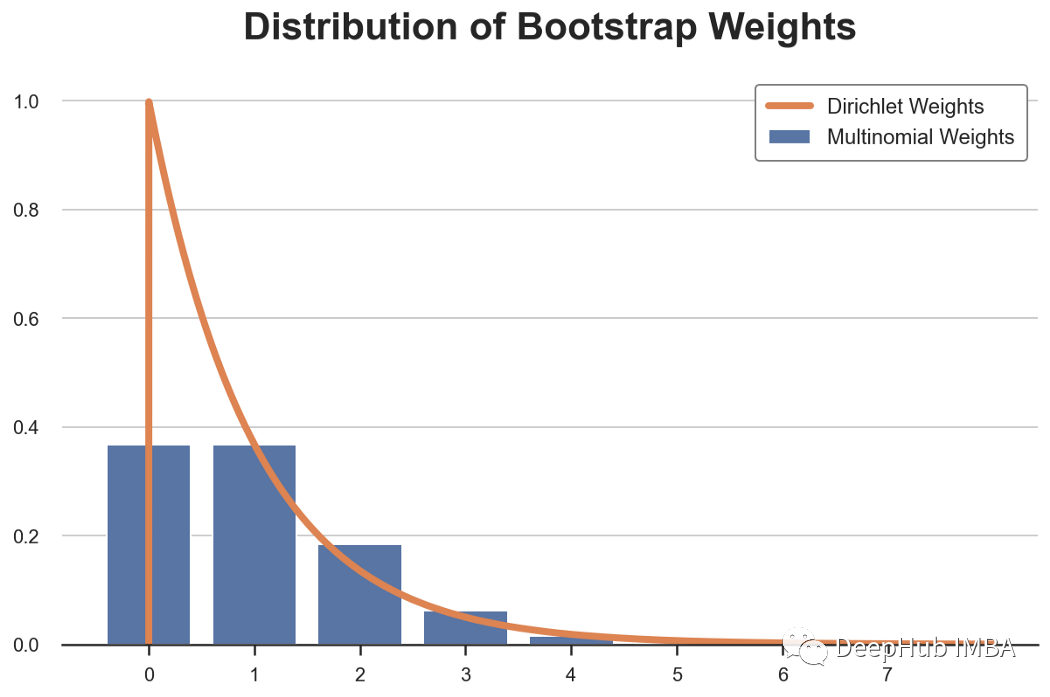bayesian_weights = np.random.dirichlet(alpha=np.ones(N), size=1) * N
np.sum(bayesian_weights)
# 10000.000000000005


 sns.histplot(bayesian_weights, color='C1', kde=True).set(title='Dirichlet Weights');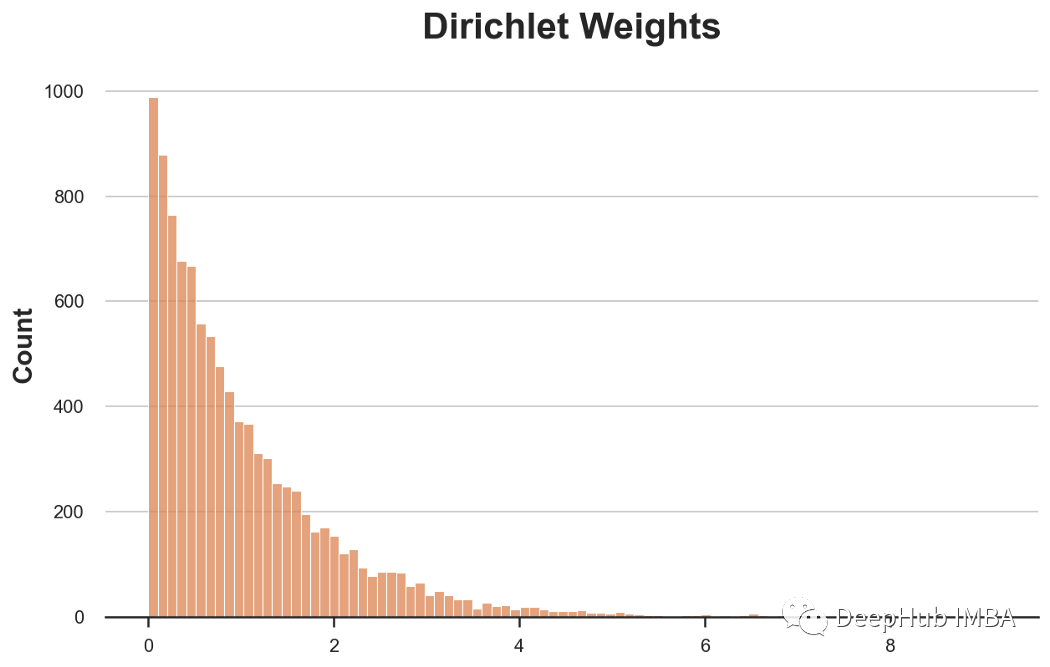α参数本质上决定被抽样的绝对概率和相对概率。增加所有观测值的α值可以减少分布的偏斜，使所有观测值具有更相似的权重。对于α→∞，所有的观测值得到相同的权重。

The distribution of the random weight vector does not have to be restricted to the Diri(l, … , 1). Later investigations found that the weights having a scaled Diri(4, … ,4) distribution give better approximations (Tu and Zheng, 1987)

 bayesian_weights2 = np.random.dirichlet(np.ones(N) * 4, 1) * N
sns.histplot(bayesian_weights, color='C1')
sns.histplot(bayesian_weights2, color='C2').set(title='Comparing Dirichlet Weights');
plt.legend([r'$\alpha = 1$', r'$\alpha = 4$']);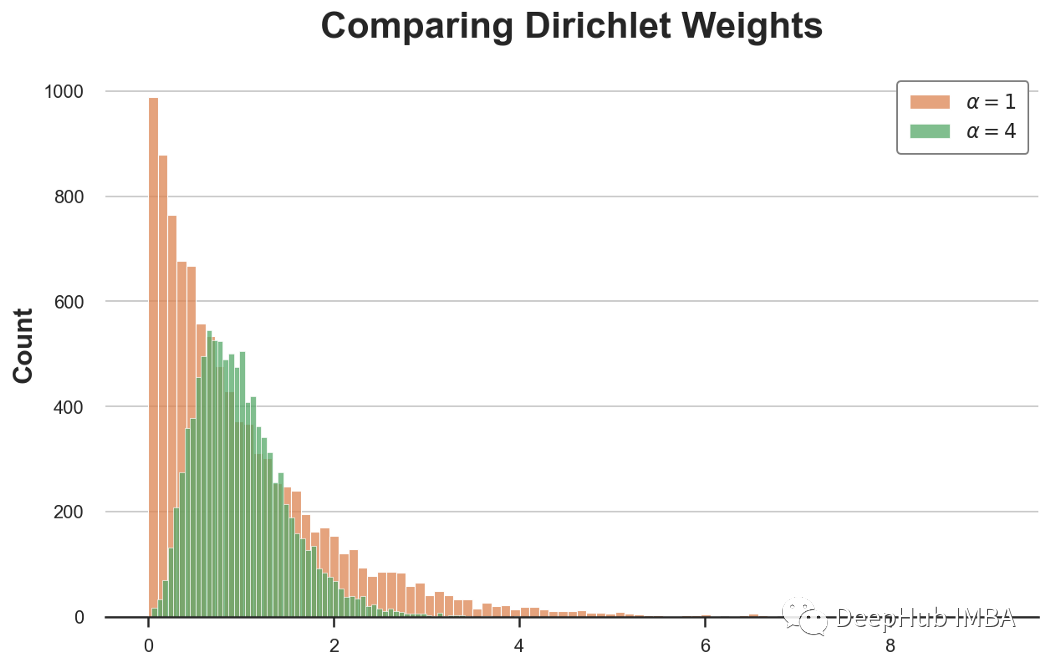## 例子

1、偏态分布的均值

 np.random.seed(2)
X = pd.Series(np.random.pareto(2, 100))
sns.histplot(X);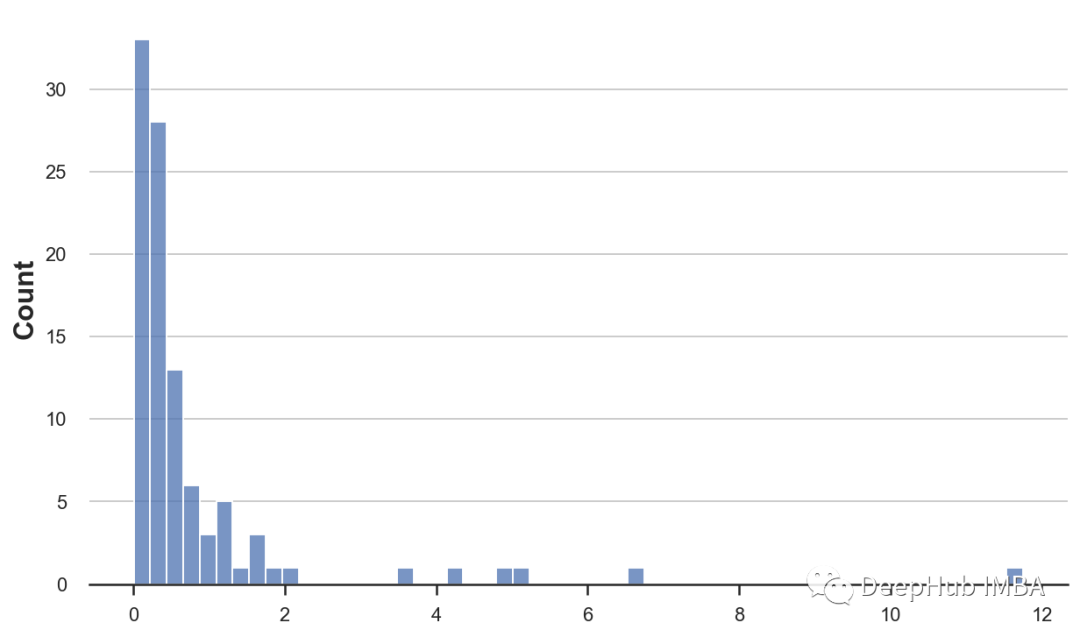def classic_boot(df, estimator, seed=1):
df_boot = df.sample(n=len(df), replace=True, random_state=seed)
estimate = estimator(df_boot)
return estimate


 def bayes_boot(df, estimator, seed=1):
np.random.seed(seed)
w = np.random.dirichlet(np.ones(len(df)), 1)
result = estimator(df, weights=w)
return result


 from joblib import Parallel, delayed

def bootstrap(boot_method, df, estimator, K):
r = Parallel(n_jobs=8)(delayed(boot_method)(df, estimator, seed=i) for i in range(K))
return r


 def compare_boot(df, boot1, boot2, estimator, title, K=1000):
s1 = bootstrap(boot1, df, estimator, K)
s2 = bootstrap(boot2, df, estimator, K)
df = pd.DataFrame({'Estimate': s1 + s2,
'Estimator': ['Classic']*K + ['Bayes']*K})
sns.histplot(data=df, x='Estimate', hue='Estimator')
plt.legend([f'Bayes:   {np.mean(s2):.2f} ({np.std(s2):.2f})',
f'Classic: {np.mean(s1):.2f} ({np.std(s1):.2f})'])
plt.title(f'Bootstrap Estimates of {title}')


 compare_boot(X, classic_boot, bayes_boot, np.average, 'Sample Mean')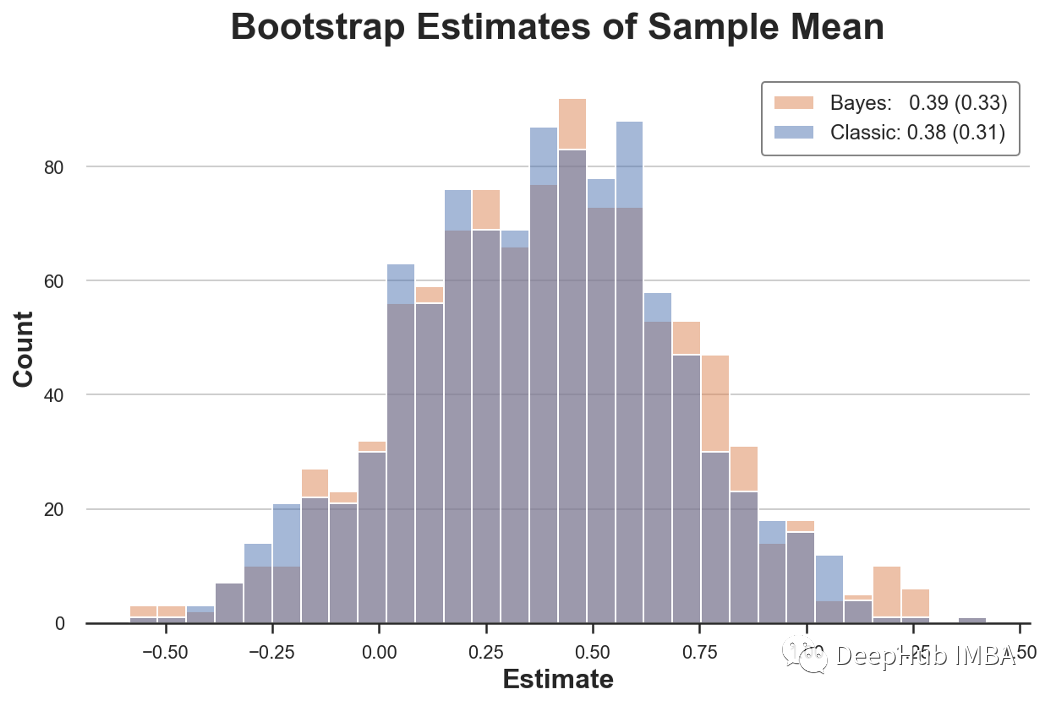import time

def compare_time(df, boot1, boot2, estimator, K=1000):
t1, t2 = np.zeros(K), np.zeros(K)
for k in range(K):

# Classic bootstrap
start = time.time()
boot1(df, estimator)
t1[k] = time.time() - start

# Bayesian bootstrap
start = time.time()
boot2(df, estimator)
t2[k] = time.time() - start

print(f"Bayes wins {np.mean(t1 > t2)*100}% of the time (by {np.mean((t1 - t2)/t1*100):.2f}%)")


 Bayes wins 97.8% of the time (by 66.46%)


2、没有权重怎么办？也没问题

 def twolv_boot(df, estimator, seed=1):
np.random.seed(seed)
w = np.random.dirichlet(np.ones(len(df))*4, 1)
df_boot = df.sample(n=len(df)*10, replace=True, weights=w, random_state=seed)
result = estimator(df_boot)
return result


 np.random.seed(1)
X = pd.Series(np.random.normal(0, 10, 1000))
compare_boot(X, classic_boot, twolv_boot, np.median, "Sample Median")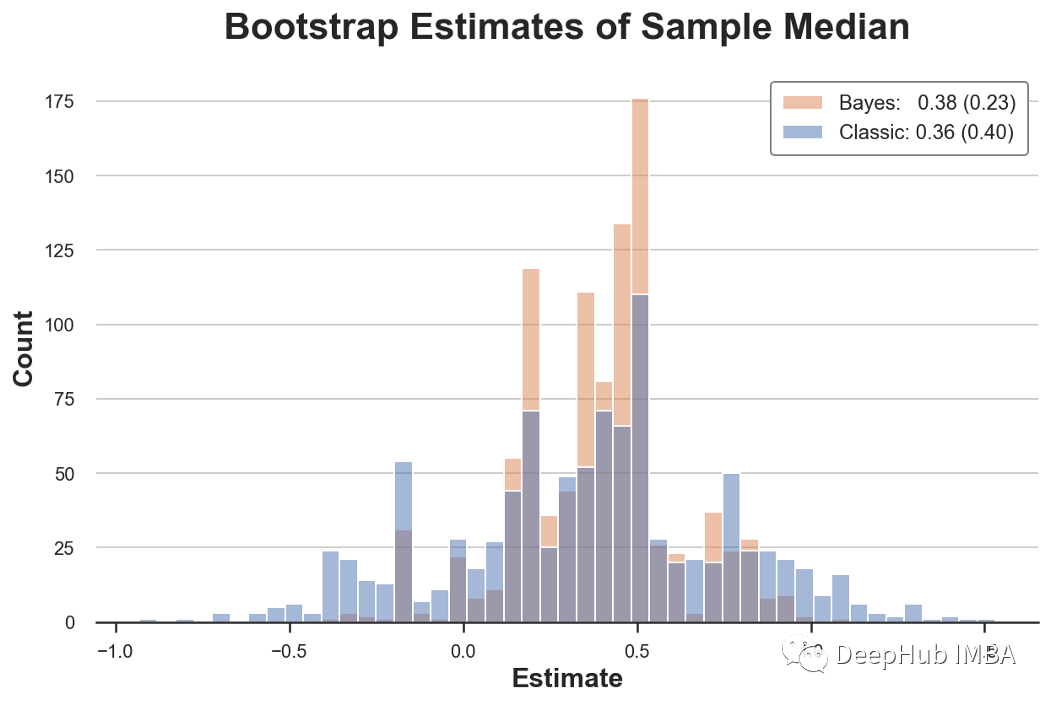3、逻辑回归和离群值

 N = 100
np.random.seed(1)
x = np.random.normal(0, 1, N)
y = np.rint(np.random.normal(x, 1, N) > 2)
df = pd.DataFrame({'x': x, 'y': y})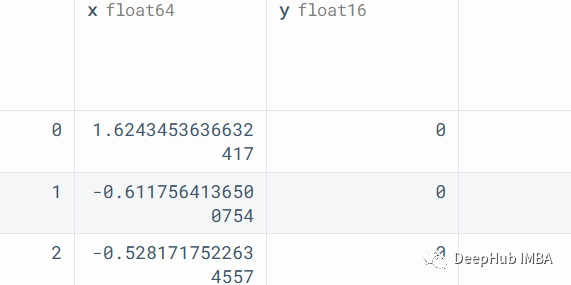smf.logit('y ~ x', data=df).fit(disp=False).summary().tables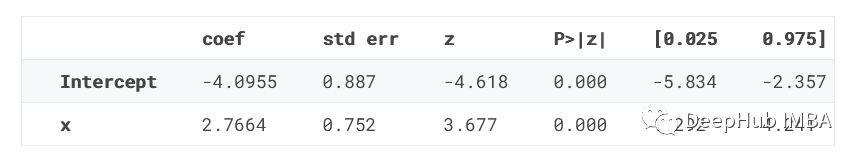estimate_logit = lambda df: smf.logit('y ~ x', data=df).fit(disp=False).params
for i in range(1000):
try:
classic_boot(df, estimate_logit, seed=i)
except Exception as e:
print(f'Error for bootstrap number {i}: {e}')


 Error for bootstrap number 92: Perfect separation detected, results not available
Error for bootstrap number 521: Perfect separation detected, results not available
Error for bootstrap number 545: Perfect separation detected, results not available
Error for bootstrap number 721: Perfect separation detected, results not available
Error for bootstrap number 835: Perfect separation detected, results not available


4、使用Treated Units进行回归

 N = 100
np.random.seed(1)
x = np.random.binomial(1, 5/N, N)
y = np.random.normal(1 + 2*x, 1, N)
df = pd.DataFrame({'x': x, 'y': y})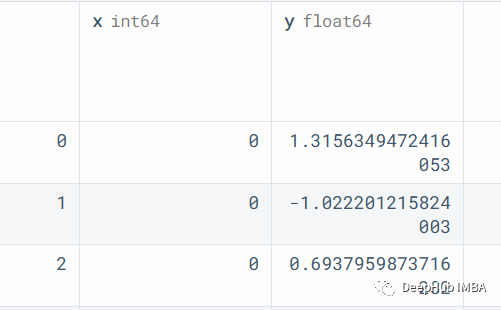estimate_beta = lambda df, **kwargs: smf.wls('y ~ x', data=df, **kwargs).fit().params
compare_boot(df, classic_boot, bayes_boot, estimate_beta, "beta")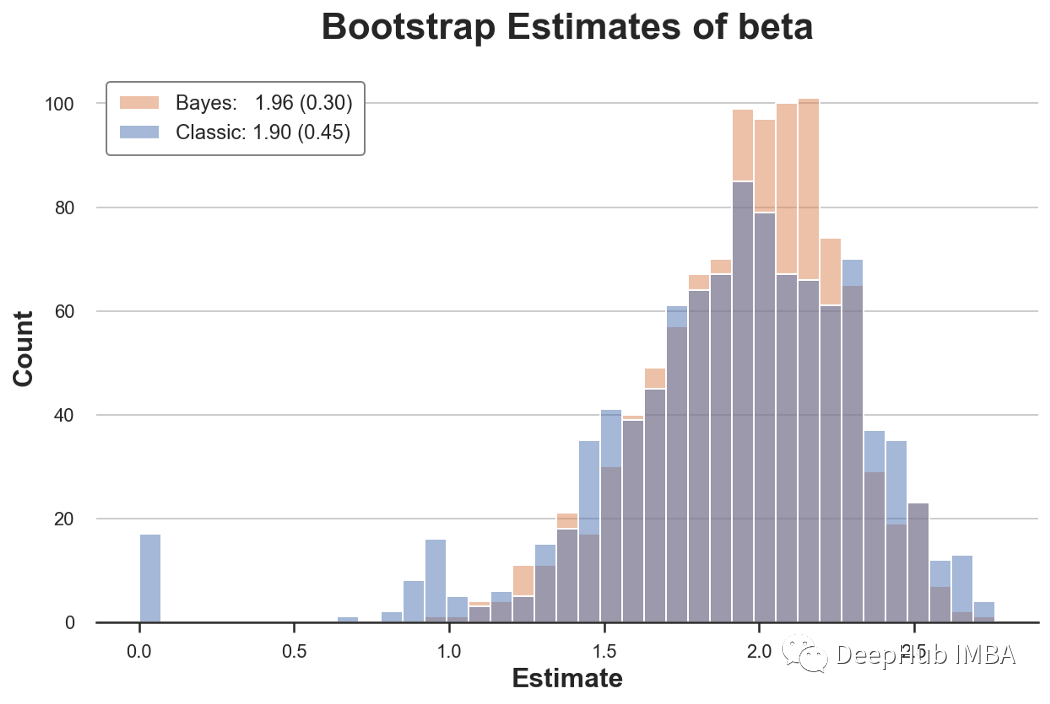## 总结

 B. Efron Bootstrap Methods: Another Look at the Jackknife (1979), The Annals of Statistics.

 D. Rubin, The Bayesian Bootstrap (1981), The Annals of Statistics.

 A. Lo, A Large Sample Study of the Bayesian Bootstrap (1987), The Annals of Statistics.

 J. Shao, D. Tu, Jacknife and Bootstrap (1995), Springer.

https://github.com/matteocourthoud/Blog-Posts/blob/main/notebooks/bayes_boot.ipynb

### “贝叶斯自举法Bayesian Bootstrap”的评论:

##### 关于作者##### Deephub